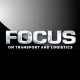# Talking power“We’ve all been there. Your vehicle won’t start, so you call passers-by to apply a combined force to give you a push-start. Two people turn up, and within a brief amount of time their combined energy has worked. The car starts and the engine’s torque provides a powerful getaway,” writes JAKE VENTER.

In everyday speech the italicised words are applied fairly loosely, but in scientific terms each has a precise meaning. When a force is applied to an object, the result depends both on the size of the object and the size of the force. If the force is big enough to cause the object to move, the result is work. Work done is equal to the force applied to an object multiplied by the distance the object is moved in the direction of the force. Force applied is measured in newtons, with one newton being the force pressing down on the palm of your hand when you hold a 100 gram apple.

In our example, if the total force applied by the pushers is 200 newtons and the car starts after being pushed 20 metres, then the work done is equal to 200 multiplied by 20, which is 4 000 newton metres (Nm). Work is measured in joules (J), with one joule being equal to one newton metre (J = Nm). In the case of our example, therefore, the amount of work done is 4 000 J. Because energy is defined as the potential for doing work, the total energy expended by the pushers in our example is also 4 000 J.

If we assume that the two pushers in our example are equally strong, each has expended 2 000 J to start the car. Now we need to determine how they can replenish that energy before continuing on their way. One possibility might be to drink some juice. In our example, each pusher is offered a small mouthful   from a bottle on which there is a label stating that its contents contain 200 000 J of energy. If each of our pushers swallows one hundredth of the bottle of juice, his energy level before expending 2 000 J on starting the car will have been restored.

Next, we may want to calculate how much power each pusher expended over the distance it took to push-start the car. Power, measured in watts (W), is defined as work done divided by the time taken. In our example, if our helpers take ten seconds to push-start the car, the work done is 2 000 divided by ten or 200 W per person. This is 0.2 kilowatts (kW) of power: well within the capability of an average adult.

But what about engines, you might ask? Because engines rotate, our definitions have to be modified. All motion, even what appears to be the aimless fluttering of a butterfly, can be studied mathematically by being broken down into a combination of straight-line and rotational components.

The rotational equivalent of a straight-line force is a torque. An easy way to explain this concept is to look at what happens when you close a door. The effect of the force you apply depends not only on the size of that force, but also on how far the point of application is from the hinge. You’ll soon discover that a small force at some distance from the hinge has the same effect as a larger force close to the hinge. Neither the size of the force nor its distance from the hinge have much meaning on their own, but when combined they produce a meaningful quantity of rotational motion known as torque.

Torque is defined as equal to the force applied multiplied by the perpendicular distance from that force to the hinge line. This is the line around which rotation takes place. Just like work, torque is measured in Nm. However, in rotational motion Nm is not equal to J because the distance involved is at right-angles or 90o to the force; in the case of work, the distance involved is parallel to the force. The rotational equivalent of distance is the angle turned through, so that in rotational motion work done is equal to the torque applied multipled by the angle turned through.

When evaluating a vehicle’s performance, the torque or pulling power – as shown by the torque curve – is an important indicator of engine capability. If an engine develops a significant amount of torque over a wide speed range, the driver can travel in higher gears most of the time. If, on the other hand, high torque values are limited to certain speeds, the driver will have to change gear frequently.

*This is the first in a two-part series. Part 2 will follow in next month’s issue of FOCUS.